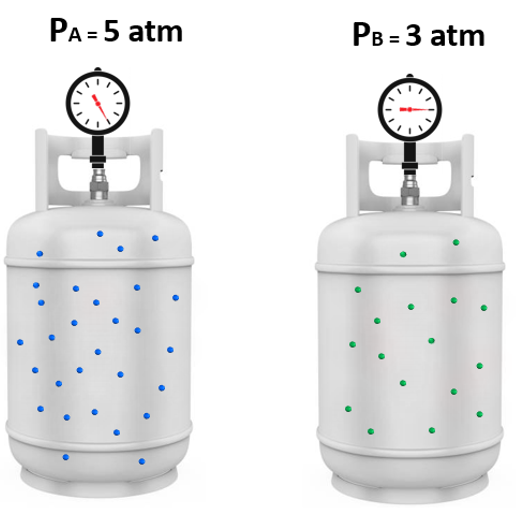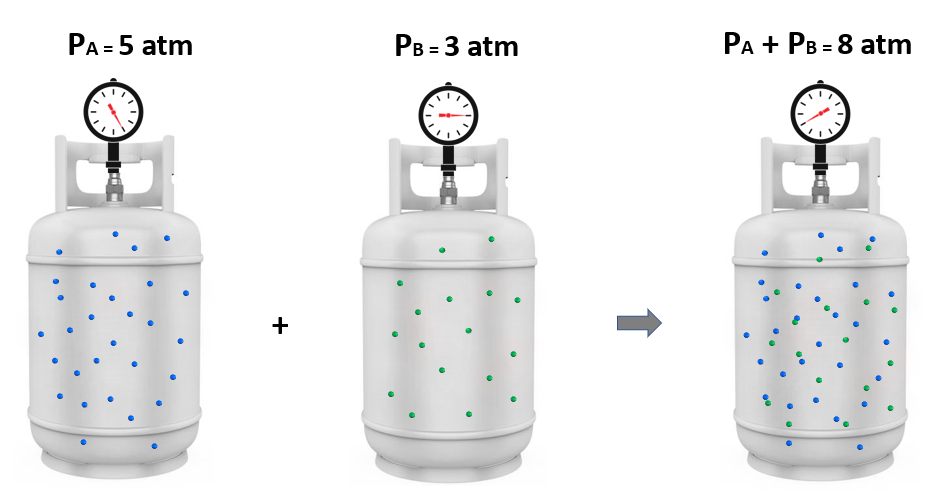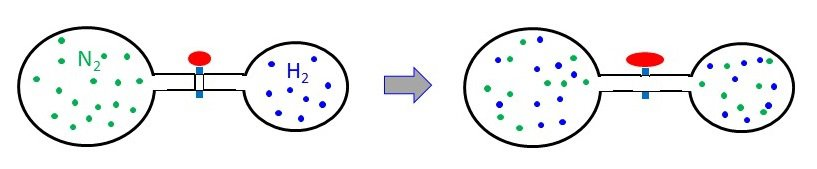## General Chemistry

To understand the partial pressures, let’s suppose a certain amount of gas “A” is added to a tank and exerts 5 atm pressure, and in another tank with gas “B” the pressure is 3 atm:Now, it turns out that if we mix these amounts of gas A and B together, the total pressure is 8 atm. And this intuitive observation was first formulated by John Dalton and is known as the Dalton’s law of partial pressures.

It states that the total pressure of a gas mixture is equal to the sum of the pressures that each gas would exert if it were present alone:The individual pressure of each gas component is called a partial pressure, so the total pressure is the sum of the partial pressures:

PTotal = PA + PB

This formula is true for any number of gas types in the, and in a more general way, we can write:

PTotal = P1 + P2 + … + Pn

For example,

What is the partial pressure of nitrogen in a mixture of N2, SO2, and CO2 that has a total pressure of 6.84 atm? The partial pressure of SO2 is 2.10 atm, and the partial pressure of COis 1.74 atm.

Solution: The total pressure is the sum of the partial pressures of all the gasses in the mixture:

PT = P(N2) + P(SO2) + P(CO2) = 6.84 atm

P(N2) = P– [P(SO2) + P(CO2)]

P(N2) = 6.84 atm – [2.10 atm + 1.74 atm]

P(N2) = 3.00 atm

Another example,

13.2 grams of COand 6.00 grams of He are mixed in a 4.00 L container at 300. K. Calculate the partial pressure of both gases and the total pressure of the mixture.

Solution: First, we need to determine the moles of the gases and then use them in the ideal gas law equation to calculate the pressure of each gas (partial pressure):

To calculate the moles, we need to do the grams-to-moles conversion based on the molar mass of each gas:

n (CO2) = 13.2 g/44.0 g/mol = 0.300 mol

n (He) = 6.00 g/4.00 g/mol = 1.50 mol

${\rm{P}}\;{\rm{ = }}\;\frac{{{\rm{nRT}}}}{{\rm{V}}}$

${\rm{P}}\;{\rm{(C}}{{\rm{O}}_{\rm{2}}}{\rm{)}}\;{\rm{ = }}\;\frac{{{\rm{nRT}}}}{{\rm{V}}}$

${\rm{P}}\;{\rm{(C}}{{\rm{O}}_{\rm{2}}}{\rm{)}}\;{\rm{ = }}\;\frac{{{\rm{0}}{\rm{.300}}\;\cancel{{{\rm{mol}}}}{\rm{ \times }}\;{\rm{0}}{\rm{.08206}}\;\cancel{{\rm{L}}}\;{\rm{atm}}\;\cancel{{{{\rm{K}}^{{\rm{ – 1}}}}}}\;{\rm{mo}}{{\rm{l}}^{{\rm{ – 1}}}}\;{\rm{300}}{\rm{.}}\;\cancel{{\rm{K}}}}}{{{\rm{4}}{\rm{.00}}\;\cancel{{\rm{L}}}}}\; = \;{\rm{1}}{\rm{.85}}\;{\rm{atm}}$

${\rm{P}}\;{\rm{(He)}}\;{\rm{ = }}\;\frac{{{\rm{1}}{\rm{.50}}\;\cancel{{{\rm{mol}}}}{\rm{ \times }}\;{\rm{0}}{\rm{.08206}}\;\cancel{{\rm{L}}}\;{\rm{atm}}\;\cancel{{{{\rm{K}}^{{\rm{ – 1}}}}}}\;{\rm{mo}}{{\rm{l}}^{{\rm{ – 1}}}}\;{\rm{300}}{\rm{.}}\;\cancel{{\rm{K}}}}}{{{\rm{4}}{\rm{.00}}\;\cancel{{\rm{L}}}}}\; = \;{\rm{9}}{\rm{.23}}\;{\rm{atm}}$

These are the partial pressure of CO2 and He, so to find the total pressure, we add them up:

Ptotal = 1.85 + 9.23 = 11.1 atm

Alternatively, since Dalton’s law applies to any gas mixture (as long as they keep the ideal behavior), the identity of the gases does not matter, and we can add the moles together first, and use the total number of moles in the ideal gas law equation. In this case, instead of the partial pressures, we will get the total pressure of the mixture.

n (total moles) = 1.50 + 0.300 = 1.80 mol

${\rm{P}}\;{\rm{(total)}}\;{\rm{ = }}\;\frac{{{\rm{1}}{\rm{.80}}\;\cancel{{{\rm{mol}}}}{\rm{ \times }}\;{\rm{0}}{\rm{.08206}}\;\cancel{{\rm{L}}}\;{\rm{atm}}\;\cancel{{{{\rm{K}}^{{\rm{ – 1}}}}}}\;{\rm{mo}}{{\rm{l}}^{{\rm{ – 1}}}}\;{\rm{300}}{\rm{.}}\;\cancel{{\rm{K}}}}}{{{\rm{4}}{\rm{.00}}\;\cancel{{\rm{L}}}}}\; = \;{\rm{11}}{\rm{.1}}\;{\rm{atm}}$

Now, the question is how can we determine the partial pressures from here? And for this, we use the percentage of each gas, which, is formulated as the mole fraction. We keep this for the next article, where we will discuss the relationship between the mole fraction and the partial pressure of the gas.

Check Also

#### Practice

1.

A 3.00-L bulb containing N2 at 1.80 atm pressure is connected to a 2.00-L bulb filled with H2 at 3.50 atm pressure. What is the final pressure of the system when the valve is opened?PN2 = 1.80 atm               PH2 = 3.50 atm                                             Ptotal = ? atm

2.48 atm

Solution

### 2 thoughts on “Dalton’s Law of Partial Pressures”

1.Thank so kindly for sharing such great knowledge in chemistry. Kudos

•